Start typing, then use the up and down arrows to select an option from the list.## General Chemistry

Learn the toughest concepts covered in Chemistry with step-by-step video tutorials and practice problems by world-class tutors

19. Chemical Thermodynamics

Entropy is the disorder or chaos associated with a system’s inability to convert thermal energy into mechanical work.

Entropy and Spontaneity
1
concept

## The 2nd and 3rd Laws of Thermodynamics1m
Play a video:
Hey, guys, In this new video, we're gonna take a look at a new variable, the variable of entropy, and we're gonna see how does the entropy relate to the spontaneity of a chemical reaction? Now, what we're gonna say here is we're going to say that the second law of thermodynamics states that molecular systems tend to move spontaneously toe a state of maximum disorder or randomness. Now, what does this mean? This means that the universe does not want to get ordered. Doesn't wanna come together in a nice little package. The universe naturally wants to go into a chaotic mode. Everything is spinning out of control. Everything is breaking down, becoming less ordered. This is the natural process of our universe. So simply put, the second law says that the universe is going to shit. Okay, so just think of it like that. The universe is getting crappy. Er, by the day, this is the natural state of the universe. This is all the second law of thermodynamics is saying now. On the opposite end of this, the third Law of thermodynamics says that at absolute zero, absolute zero is zero kelvin at absolute zero. The entropy of all objects is equal to zero. So basically, everything is always gonna be in disorder. Always trying to get more and more disordered. Unless it's at zero. Kelvin at zero. Calvin, entropy or chaos will stop. And remember, this is the third law of thermodynamics. So it goes against the second law of thermodynamics. Now we're gonna say that again. Entropy is chaos, disorder, randomness, shiftiness, whatever you want to say, it's called, and the variable we use for entropy is s.

The 2nd Law of Thermodynamics states systems (chemical reactions) spontaneously move to state of disorder.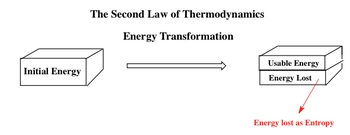2
concept

## Entropy & Phase Changes1m
Play a video:
Now let's think about this in a molecular image wise. So we're gonna say in general, as we move from a solid to a liquid toe, a gas, what's happening to the molecules of any compound? So remember, a solid all the molecules are tightly packed together on top of each other. Then, as we become a liquid, the molecules air still around each other. But because it's a liquid there, naturally sliding over each other, moving around one another. And then as they become a gas, they become very spaced out, so they're bouncing all over the place. So we're gonna say in this natural progression, when we go from solid liquid to gas, we're gonna say entropy or chaos will increase because the molecules get farther and farther apart and of entropy is increasing in the sign of entropy will be positive. Now think about it in the opposite way. In the opposite way. We have gasses which are very spaced out. They condense into a liquid. Now the molecules are even closer together. They're not frozen in place yet. We haven't gotten to a solid yet, but they're sliding over one another. Then finally, when we get to a solid. They're frozen in place. So in this direction, we're gonna say that entropy decreases because order is increasing. And if entropy is decreasing, the sign of entropy will be negative.

During a phase change as our molecules grow farther apart then entropy will increase.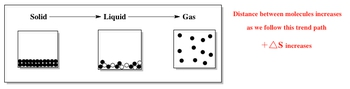During a phase change as our molecules grow closer together then entropy will decrease.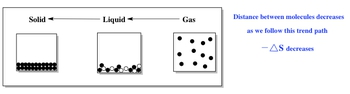3
example

## Which should have the highest molar entropy at 25oC33s
Play a video:
now taking that into consideration. Let's look at example one, it says, which should have the highest Moeller entropy at 25 degrees Celsius. So here I give you gallium and it's three different phases. We have liquid solid and gas. Now remember, we're just saying who has the highest entropy? We just said Who has the most chaos, whose molecules are most spread out. This is a very easy question. We're gonna say simply the gas phase would have the most entropy because it's the most spread out.
4
example

## Which substance has greater molar entropy4m
Play a video:
we're gonna say which substance, for example, to which substance has greater mola entropy. So this will be we're gonna compare these two, these two and these two. So within those three choices, we're gonna choose the best out of each one. So the first one we have ch four gas or CCL four liquid. Now, the way you look at these types of questions is you first look at their faces, Okay, so we first look at phases and are you going to say here is gasses have higher entropy, then liquids and liquids have higher entropy than solids. Now, if they're tied in the same phase, then we move on to complexity. And what the heck do I mean by complexity? All I'm saying is the Mawr elements that make up the compound the more entropy. So the mawr elements that make up the compound than the mawr Entropy. So, for example, if I gave you co two gas versus S eight gas, you would say that CO two is made up off three different atoms. One carbon to oxygen's. An essay is made up of eight atoms of sulfur. So it has mawr elements that make it up. So it has more entropy, so essay would have more entropy. Now, if they have the same number of elements that make them up, then we go to overall mass. And here for three, you're gonna say more mass equals more entropy. Because think of it like this. We said that entropy is chaos, destruction, disorder, randomness. So if we want to think about it, think of it as two cars on the highway. You have a little mini Cooper versus Hummer. Okay, You're gonna say the Hummer is much larger, and if it gets in an accident, it's gonna cause more destruction, more disorder. So we're gonna say the Hummer has a greater mass compared to the smaller car compared to the Mini Cooper. So just think of it. In those terms, more mass equals bigger sides equals mawr chaos that we can have So here, their different phases. So we can easily say it's the gas now for the next one. They're both gasses, so the first one doesn't help us. Let's look at the second criteria. We're gonna say complexity. Here. Both have the same number of atoms. One, there's only one neon Wonsan on so that doesn't help us. So then we move on to the third one mass. We're gonna say that Zen on on our periodic table has a higher atomic mass than neon. And because of that, Zen in has a higher entropy. Then finally, let's look at the last one. We have ch 30 h liquid versus C six h 50 h liquid. So they both have the same face again. Sold. The first rule doesn't help us. What we do next is we look at complexity. We're gonna say C six h 50 h definitely has mawr elements that make it up. So it's more complex, more moving parts mawr, chances of chaos and entropy. So we're gonna say C six h five has mawr entropy Now you could have also simply said that C six h five has a greater mass and because of that, it should have the greater entropy. So remember, Delta s is just chaos or disorder. The farther molecules are from each other in their phases than the greater the entropy. So gashes compounds have mawr entropy than liquids or solids. And we're going to say that the natural tendency of the universe is to be in disorder. So the second law of thermodynamics is saying that the universe is going to shit. Or you could just say that the second law of thermodynamics is saying that the entropy of the universe must always be greater than zero. Because if entropy is always increasing in our universe, there's no chance for it to be less than zero. Always has to be greater than zero. Just remember that for the second law of thermodynamics
Entropy and Phases

When comparing the entropy of different compounds then we must follow a set of guidelines in the following order.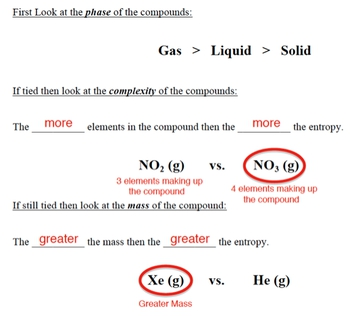5
example

## Arrange the following substances in the order of increasing entropy at 25oC4m
Play a video:
Hey guys, in this new video, we're going to continue with our discussion of entropy and the way it relates to spontaneous reactions. So let's take a look. At example, one an example. One, it says arranged the following substances in order of increasing entropy at 25 degrees Celsius. So I want in order of increasing entropy. So that means least entropy to most entropy. Now, remember, we talked about the criterias we have to look at to determine who has the most entropy. First you have to look at the faces and remember, gasses have the most, then liquids, then solids. Now, your professor might be a little crafty in terms of this. Just remember, they might use the word acquis instead of liquid. But we're gonna say right now that those words are interchangeable. Liquid is just the solvent that's dissolving the compound. Acquis means that it's dissolving within our liquid. So we're gonna basically say they're similar to one another. Next, if they have the same phase, then we look at complexity. Remember, the more elements that you have within the compound that make it up, the more complex it is, and therefore, the more entropy it has. So let's say, for example, we're looking at two compounds that had the same face. Let's say we're looking at C 286 at the gas and C three h eight propane gas. They're both gasses. So then we have to look at complexity. We're gonna say propane, which is C three h eight is made up off 11 atoms. Three carbons, eight hydrogen ethane, on the other hand, is only see two age six. It only has eight elements making it up. The more elements within your compound, the more complex it ISS and therefore the higher it's entropy. So C three h eight will be higher. Next. We're gonna say if they have the same number of atoms, then we look at mass. Are you going to say the greater the mass, the greater the entropy? Remember the whole analogy? We have two cars out of control on a highway. Who's gonna cause more damage? The Mini Cooper or the Hummer? The Hummers, much larger, has greater mass, therefore can cause greater damage. So let's take a look here. We have to put them in order. We have a gas here and we have a gas here. So those two, we expect to have the highest entropy. So because they're both gasses, we go on to the next criteria. Complexity H. I is made up of two different elements. H two is also made up of two elements, so they are tied for complexity. So the tie breaker has to be mass h. I has greater mass or h I should have greater entropy. Now that we're done with gas is we can move on to acquiesce and liquids. Here we have liquid and liquid, so we're gonna say H g is liquid and B R two is liquid. Br two has greater complexity because there's too bro means together and then murky would be next. Next we're gonna look at is Zenon Tetra Florida X E F four vs b o. They're both solids, right? So they're tied and phase. So we have to look at complexity. The one with more complexity will have greater entropy. So Zen in Tetra Florida's XY F four. That's five elements that make it up be Ailes Only two elements that make it up. So it be Xena tetra fluoride solid and then finally, barium oxide would have the least entropy because it's ah solid, which has the lowest and also it's only made up of two elements. Therefore, its complexity is much lower than XY F four. Hopefully, guys were able to follow along with these three criterias, so just remember, look at face first, then look at complexity. If that all fails, look at mass. The greater the mass, the greater the entropy. Now that we've seen this example, let's move on to example, too.
6
example

## Containers A and B have two different gases that are allowed to enter Container C3m
Play a video:
7
Problem

An ideal gas is allowed to expand at constant temperature. What are the signs of ∆H, ∆S & ∆G.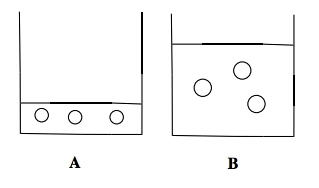Whenever a phase change occurs, first determine if bonds are broken or formed to figure out the signs of enthalpy and entropy. Afterwards, determine if the reaction is spontaneous to determine the sign of Gibbs Free energy.

8
example

## Consider the spontaneous fusion of ice at room temperature4m
Play a video:
9
Problem

Consider the freezing of liquid water at 30°C. For this process what are the signs for ∆H, ∆S, and ∆G?

If bonds are broken then the entropy of a reaction increases, but if bonds are formed then the entropy of a reaction decreases.

10
Problem

Predict the sign of ∆S in the system for each of the following processes:

a) Ag+ (aq) + Br - (aq) → AgBr (s)

b) CI2 (g) → 2 CI - (g)

c) CaCO3 (s) → CaO (s) + CO2 (g)

d) Pb (s) at 50°C → Pb (s) at 70°C

11
Problem

For each of the following reactions state the signs of ∆H (enthalpy) and ∆S (entropy):

a) Fusion of ice.

b) Sublimation of CO2

c) Vaporization of aqueous water.

d) Deposition of chlorine gas.

e) Condensation of water vapor.

Entropy and Calculations

The 2nd Law of Thermodynamics states that the entropy of the universe is always increasing and so it must always be greater than zero.

12
concept

## The Universe & Entropy1m
Play a video:
Hey, guys, In this new video, we're gonna take a look at calculating entropy for a system. Now, first of all, before we begin, just remember, we have the term system. When we say the words system, we mean that this is the portion of the universe that we're concerned with when we say system, we're really talking about the balanced chemical equation. So a system is just are balanced equation that we're looking at. Now we're saying that the second law of thermodynamics talks in terms of the system, and it says that the entropy of a system increases spontaneously. Remember, the entropy of the universe is gradually increasing. The world is getting mawr and more chaotic. Now we're going to say that we can't Onley focus on our system. Besides our system, we have our surroundings. We're gonna say our systems, plus our surroundings gives us our entropy total or our universe. Okay, so system plus surroundings gives us our total universe. Now we're gonna say thus, to calculate the total entropy change Delta s total. We use the following equation. So we're just gonna say Delta s total is the same thing as Delta s universe and that just equals Delta s of my system. Plus delta s of my surroundings. Okay, remember when we say system, we really mean reaction. So Delta s system could also mean Delta s off our reaction.

The entropy of the universe takes the look at the entropy of our system (the chemical reaction) and of the universe.

13
concept

## Total Entropy & Spontaneity1m
Play a video:
Now, we're going to say here in terms of spontaneity, we're going to say if our delta S total is greater than zero, then the reaction is spontaneous. If it's less than zero, then it's non spontaneous. And if it's equal to zero, then our reaction is simply at equilibrium. OK. So just remember that we have to take into consideration both our system and our surroundings as a whole to determine if a reaction is spontaneous overall or not. Because sometimes your delta S system may be highly spontaneous, meaning that the, it's delta S value is very positive. But if we add in the surroundings and at the end, we get a total that's negative, then overall it would be non spontaneous. Have to take into consideration both aspects our system and our surroundings.

If the entropy is greater than zero then we classify the process as spontaneous.

14
concept

## The Entropy of a Reaction5m
Play a video:
So if we take a look here, it says, calculate the standard entropy in kilojoules of a reaction at 25 degrees Celsius for the following reaction. So we have one mole of nitrogen gas, combining with three moles of hydrogen gas to give us two moles of ammonia gas as product. And here I give you the standard molar entropy of N two H two and N H three as these numbers respectively. Now any time your professor asks you to find the delta s of our system, they're really saying again, the delta s of our reaction and that is just products, the summation of products minus the summation of reactants. So products minus reactants. And remember this also goes for delta G and delta H. Sometimes you'll be asked to calculate the standard entropy delta H or the standard gives free energy. Delta G. In those cases, delta G system del delta H system are products minus reactants. So here we're just gonna say it equals we have, remember the coefficients 23 and one. So we're gonna say according to our balance equation, we have two moles of N H three, Each NH 3 is this total right here. That's our products minus our reactants. We have one mole and two, each one is 1 91.5 Jews over K times moles plus the other reactant, which is three moles of H two times 1 30.6 Jews over K times moles. Now, we can see that the moles will cancel out and our units here will be in jewels over Kelvin. So when we multiply the two times the 1 92.3, that's gonna give us 3 84.6 Jews over Kelvin minus everything inside of our reactance boxes that comes out to 5 83.3 Jews over Kelvin. Now just subtract those two that gives me -1 90 98.7 jewels over Kelvin. But remember I asked for the answer in killer Jews not in jewels. So we have to do one more conversion. We want kilojoules, one kilojoule, one kg is 10 to the three jewels and remember 10 to the three is the same thing as 1000. So jewels cancel out. So our units at the end will be kilojoules over Kelvin. So it's gonna be negative 0.1987 kilojoules over Kelvin. So that'll be our answers. Just remember if they ask you to find the standard entropy of our reaction. It's just products minus reactants. Now, one more thing we need to realize here just before we move on to the next set of videos. Just remember here, we're gonna say that N two and H two are both in their natural states. Remember your natural state means you're by yourself. You're an element by yourself. Example, carbon graphite or sodium solid or you're connected to copies of yourself such as N two or H two or S eight or P four. So if you're by yourself, you're connected to copies of yourself, that's your natural state. Now we're gonna say here, although N two and H two are both in their natural states, their delta S values are not equal to zero. Remember delta S um uh value is only equal to zero if the temperature is at absolute 00, Kelvin. Now we're gonna say that for an element in its natural state. Delta H and delta G both equal zero. So if they give us the values for N two and H two here, they both would be zero when we're looking at the delta H and their delta G values. Why? Because they're in their natural state. So I just want you guys to remember this for something in its natural state. It means it's by itself or it's connected to copies of itself. In either case, delta G and delta H equals zero. Delta S can only equal zero for those compounds if we're at zero. Kelvin, remember that's the third law of thermodynamics. Just remember that difference. And you guys can answer those types of theory questions when you see them on exams.

The entropy, enthalpy and Gibbs Free energy of a reaction is equal to products minus reactants.

15
example

## The oxidation of iron metal is given by the following reaction5m
Play a video:
16
Problem

Diethyl ether (C4H10O2, MW = 90.1 g/mol) has a boiling point of 35.6oC and heat of vaporization of 26.7 kJ/mol. What is the change in entropy (in kJ/K) when 3.2 g of diethyl ether at 35.6oC vaporizes at its boiling point?

17
concept

## Calculating Entropy7m
Play a video: